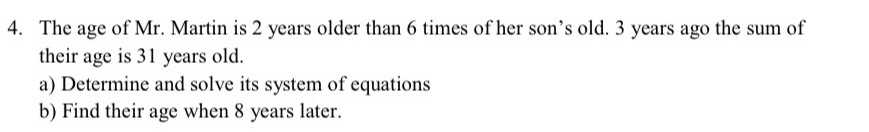### ¿Todavía tienes preguntas de matemáticas?

Pregunte a nuestros tutores expertos
Algebra
Pregunta4. The age of Mr. Martin is $$2$$ years older than $$6$$ times of her son's old. $$3$$ years ago the sum of their age is $$31$$ years old. a) Determine and solve its system of equations b) Find their age when $$8$$ years later.